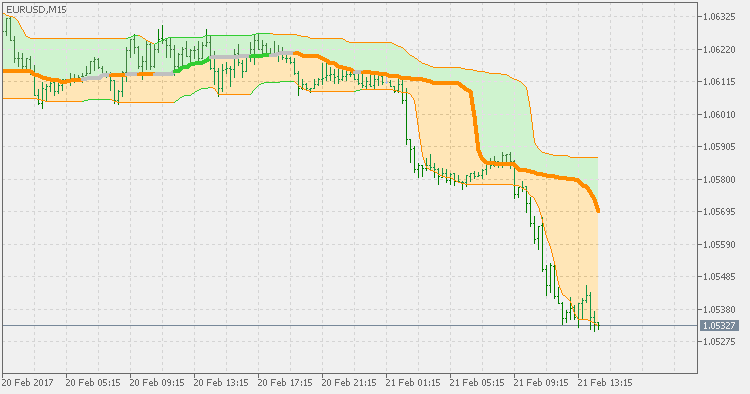# Quantile bands – 1.5 – indicator for MetaTrader 5

• Currency pairs: Any pairs, indices and metals
• Trading Time: Around the clock
• Recommended broker: icmarkets, XM
• There is already one version of quantile bands (here : https://www.mql5.com/en/code/16491) but some changes needed to be made in order to reflect the lates metatrader 5 development, and some code optimization has been done in order to keep the whole thing cleaner and more usable.

In short – Quantiles  are:

In statistics and the theory of probability, quantiles are cutpoints dividing the range of a probability distribution
into contiguous intervals with equal probabilities, or dividing the
observations in a sample in the same way. There is one less quantile
than the number of groups created. Thus quartiles
are the three cut points that will divide a dataset into four
equal-size groups (cf. depicted example). Common quantiles have special
names: for instance quartile, decile (creating 10 groups: see below for
more). The groups created are termed halves, thirds, quarters, etc.,
though sometimes the terms for the quantile are used for the groups
created, rather than for the cut points.

qQuantiles are values that partition a finite set of values into q subsets of (nearly) equal sizes. There are q − 1 of the q-quantiles, one for each integer k satisfying 0 < k < q. In some cases the value of a quantile may not be uniquely determined, as can be the case for the median
(2-quantile) of a uniform probability distribution on a set of even
size. Quantiles can also be applied to continuous distributions,
providing a way to generalize rank statistics to continuous variables. When the cumulative distribution function of a random variable is known, the q-quantiles are the application of the quantile function (the inverse function of the cumulative distribution function) to the values {1/q, 2/q, …, (q − 1)/q}.

The indicator middle line is not based
on the slope of the middle quantile. Instead it is a combination of the
two outer quantiles. When both outer quantiles are showing the same
“trend” the color of the middle quantile is the same as the outer
quantiles, otherwise it is “undetermined” (grey, if you use default
settings).• How to install MT5 indicator?
• Related Posts
• 1. On the desktop, double-click to open the MT45 trading platform software you installed.
• 2. Click on “File” in the upper left corner – “Open Data Folder”, double-click the MQL5 folder, and double-click the Indicators folder
• 3. Copy the metrics you need to install to the Indicators folder
• 4. Open MT5, find “Navigator” in the display menu, then click “Technical Indicators” of “Navigator” and right click to refresh, you will see the indicator just copied.
• 5. In the “Technical Indicators” of “Navigator”, find the indicator file just installed, double click or click manually to the chart window